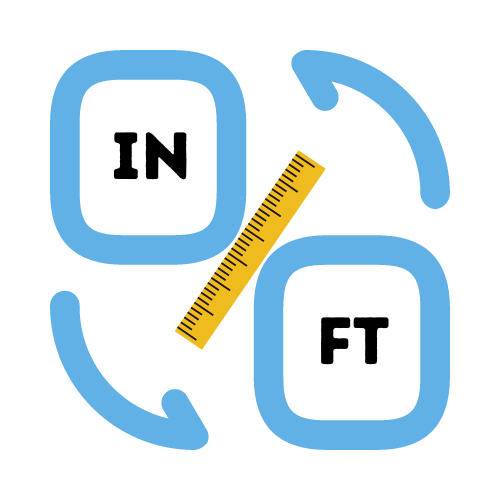# Inches to Feet Conversion Calculator

Total length:

## Why do we calculate in feets and inches?

Inches, feet and yards are units of linear measurements. Inches are commonly used in sewing, tailoring, carpentry, construction, etc. Inches are also used in printing and display. They are frequently used to measure the height of a person, diameter of a pipe, width of a carpet, thickness of a sheet, height of a building/structure, etc.

Measuring length in feet is something that originated with our ancestors, dating back to around 2575 BC. Historically, the "foot" measurement was used by the Greeks, Romans, Chinese, French and early English civilizations.

## How many inches are in a foot?

Each foot has a length of 12 inches. Feet and inches are widely used as a unit of measurement in the United States and many other countries in the world.

When we talk about the length of a room or a house, we usually use the word "foot" when referring to feet and inches. The same terminology is used when describing the height of a person.

However, we normally shorten the description of height. For example: we would say 5'10, which is understood to be 5 feet and 10 inches.

## How to use the Inches to Feet Length Calculator

Enter the length in inches and click the calculate button. The calculator will instantly convert the length into feet.

If the value in inches is not divisible by 12 then the result will show the result with 2 decimal places.

## How do you convert inches to feet?

We already know that there are 12 inches in a foot, so we can divide 1 by 12 and get the inche in equivalent decimal length (`1/12 = 0.083333 feet`). Now we know that an inch is equal to the length of 0.0833 feet.

Since we know the corresponding length for each unit, we can calculate the length in either feet or inches quite easily. An example conversion of foot to inches: 10 foot to inches can be calculated as `10 * 12 = 120 inches`.

What about converting from inches to feet? To do that we simply take the inches value and multiply the number by the decimal equivalent of an inch like we discussed above. Let's take the same example we did above but reverse the process. To convert 120 inches to feet we simply do `120 * 0.083333 = 10 feet`.